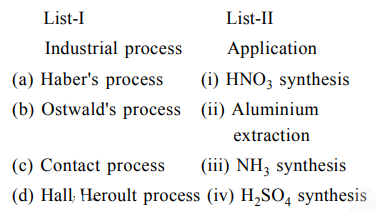# Match List-I with List-II :`
Question:

Match List-I with List-II :Choose the correct answer from the options given below:

1. (a)-(ii), (b)-(iii), (c)-(iv), (d)-(i)

2. (a)-(iii), (b)-(iv), (c)-(i), (d)-(ii)

3. (a)-(iii), (b)-(i), (c)-(iv), (d)-(ii)

4. (a)-(iv), (b)-(i), (c)-(ii), (d)-(iii)

Correct Option: , 3

Solution:

(a) Haber's process is used for $\mathrm{NH}_{3}$ synthesis.

(b) Ostwald's process is used for $\mathrm{HNO}_{3}$ synthesis.

(c) Contact process is used for $\mathrm{H}_{2} \mathrm{SO}_{4}$ synthesis.

(d) In Hall-Heroult process, electrolytic reduction of impure alumina can be done.

(Aluminium extraction)